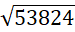# Quiz Discussion

The least perfect square, which is divisible by each of 21, 36 and 66 is:

Course Name: Quantitative Aptitude

• 1] 213444
• 2] 214344
• 3] 214434
• 4] 231444
##### Solution
No Solution Present Yet

#### Top 5 Similar Quiz - Based On AI&ML

Quiz Recommendation System API Link - https://fresherbell-quiz-api.herokuapp.com/fresherbell_quiz_api

# Quiz
1
Discuss

$$\root 3 \of {\sqrt {0.000064} } = ?$$

• 1] 0.02
• 2] 0.2
• 3] 2
• 4] None of these
##### Solution
2
Discuss

The digit in the units place in the square root of 15876 is

• 1]

8

• 2]

6

• 3]

4

• 4]

2

##### Solution
3
Discuss

If $$\sqrt 5 = 2.236,$$   then the value of $$\frac{{\sqrt 5 }}{2} - \frac{{10}}{{\sqrt 5 }} + \sqrt {125}$$   is equal to :

• 1] 5.59
• 2] 7.826
• 3] 8.944
• 4] 10.062
##### Solution
4
Discuss

Given $$\sqrt 2 = 1.414.$$   Then the value of $$\sqrt 8$$  + $$2\sqrt {32}$$  -  $$3\sqrt {128}$$  + $$4\sqrt {50}$$   is = ?

• 1] 8.426
• 2] 8.484
• 3] 8.526
• 4] 8.876
##### Solution
5
Discuss

The value of $$\sqrt {0.01} { \text{ + }} \sqrt {0.81} { \text{ + }} \sqrt {1.21} { \text{ + }} \sqrt {0.0009}$$  is = ?

• 1] 2.03
• 2] 2.1
• 3] 2.11
• 4] 2.13
##### Solution
6
Discuss=

• 1]

232

• 2]

123

• 3]

432

• 4]

543

##### Solution
7
Discuss

If a = 0.1039, then the value of $$\sqrt {4{a^2} - 4a + 1} + 3a$$     is:

• 1] 0.1039
• 2] 0.2078
• 3] 1.1039
• 4] 2.1039
##### Solution
8
Discuss

If √x+x/y  = x√x/y  where x and y are positive real numbers, then y is equal to ?

• 1]

x + 1

• 2]

x - 1

• 3]

x2 + 1

• 4]

x2 - 1

##### Solution
9
Discuss

If $$\sqrt 5 = 2.236{ \text{,}}$$   then the value of $$\frac{1}{{\sqrt 5 }}$$ is = ?

• 1] 0.367
• 2] 0.447
• 3] 0.745
• 4] None of these
##### Solution
10
Discuss

How many perfect squares lie between 120 and 300 ?

• 1] 5
• 2] 6
• 3] 7
• 4] 8
# Quiz